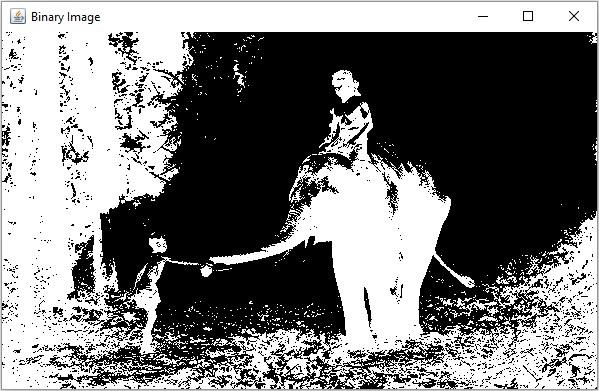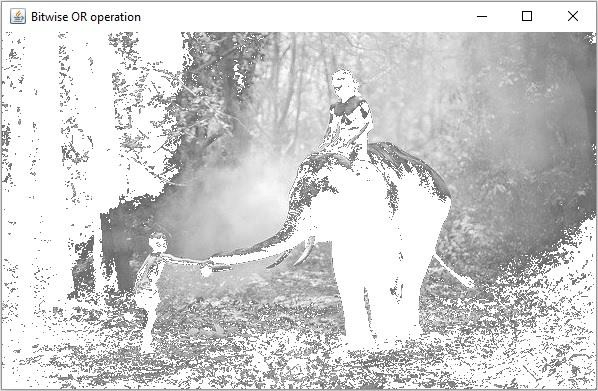# How to perform Bitwise OR operation on two images using Java OpenCV?

You can compute bitwise or between two images using the bitwise_or() method of the org.opencv.core.Core class.

This method accepts three Mat objects representing the source, destination and result matrices, calculates the bitwise disjunction of each element in the source matrices and stores the result in the destination matrix.

## Example

In the following Java example, we are converting an image into a binary and grayscale and calculating the bitwise disjunction of the results.

import org.opencv.core.Core;
import org.opencv.core.Mat;
import org.opencv.highgui.HighGui;
import org.opencv.imgcodecs.Imgcodecs;
import org.opencv.imgproc.Imgproc;
public class BitwiseORExample {
public static void main(String args[]) throws Exception {
String file ="D://images//elephant.jpg";
HighGui.imshow("Grayscale Image", src);
//Creating an empty matrix to store the results
Mat dst = new Mat(src.rows(), src.cols(), src.type());
Mat threshold = new Mat(src.rows(), src.cols(), src.type());
//Converting the gray scale image to binary image
Imgproc.threshold(src, threshold, 100, 255, Imgproc.THRESH_BINARY_INV);
HighGui.imshow("Binary Image", threshold);
//Applying bitwise Or operation
Core.bitwise_or(src, threshold, dst);
HighGui.imshow("Bitwise OR operation", dst);
HighGui.waitKey();
}
}

## Input Image## Output

On executing, the above program generates the following windows −

Gray Scale Image −Binary Image −Bitwise Or −